## Hypervalency: Is it real?The Wikipedia page on hypervalent compounds reveals that the concept is almost as old as that of normally valent compounds. The definition there,  is “a molecule that contains one or more main group elements formally bearing more than eight electrons in their valence shells” (although it could equally apply to e.g. transition elements that would contain e.g. more than 18 electrons in their valence shell). The most extreme example would perhaps be of iodine (or perhaps xenon). The normal valency of iodine is one (to formally complete the octet in the valence shell) but of course compounds such as IF7 imply the valency might reach 7 (and by implication that the octet of electrons expands to 14). So what of IF7? Well, there is a problem due to the high electronegativity of the fluorine. One could argue that the bonds in this molecule are ionic, and hence that the valence electrons really reside in lone pairs on the F. Thus the apparently hypervalent PF5 could be written PF4+…F, in which case the P is not really hypervalent after all. We need a compound with un-arguably covalent bonds. Well, what about IH7? One might probably still argue about ionicity (for example H+…IH6) but that puts electrons on I and not H, and hence does not change any hypervalency on the iodine. Surely, if hypervalency is a real phenomenon, it should manifest in IH7?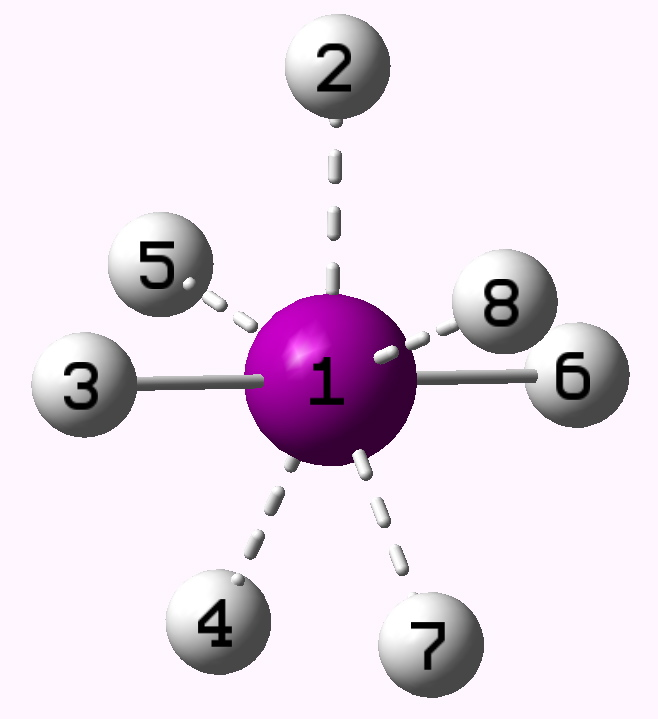IH7. A true hypervalent molecule? Click for 3D

A reasonably high level calculation (B3LYP/Def2-TZVPP with pseudopotential on I to absorb relativistic effects) shows the molecule to be a minimum, with D5h symmetry. An NBO analysis shows 46 core (i.e. non-valence) electrons. These arise from a Kr core (=36) plus a filled iodine 5d shell of 10 electrons. So, only the 5s/5p orbitals can be used for valence bonding. The total minimal basis which one can construct valence molecular orbitals from is thus I 5s, 5p and H 1s, a total of eleven AOs, into which 14 electrons must pair up into 7 doubly-occupied molecular orbitals. Now, the real issue is whether this occupancy corresponds to seven I-H covalent single bonds, each with a two-electron Lewis pair. If so, the molecule is hypervalent! The occupied MOs are shown below.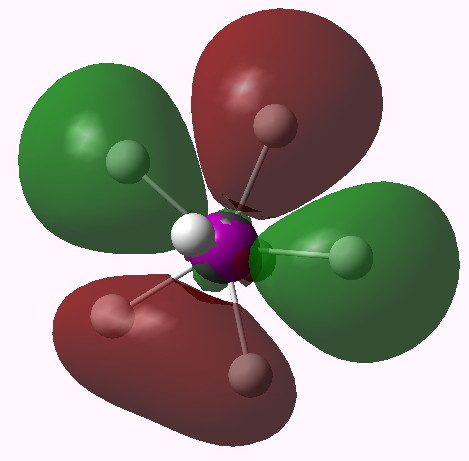MO 16 (E2'). Click for 3D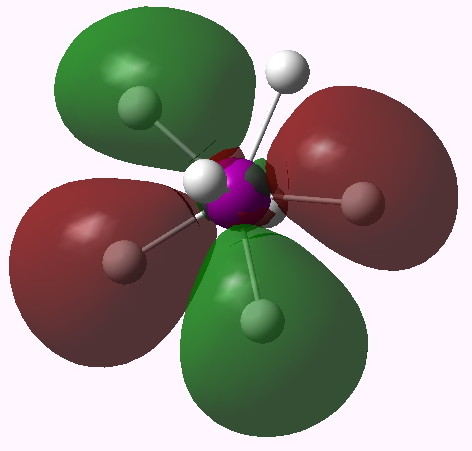MO 15 (E2')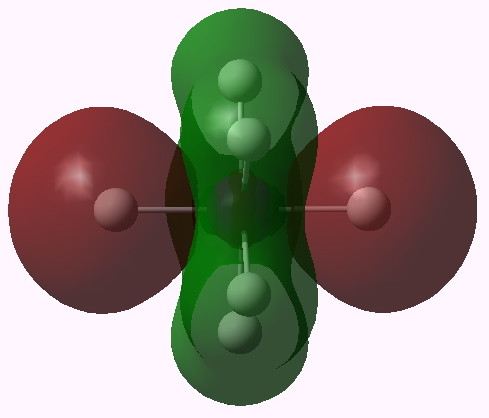MO 14 (A1')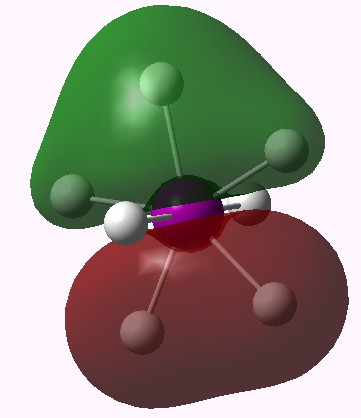MO 13 (E1')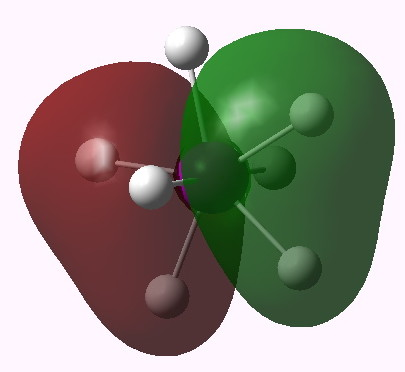MO 12 (E1')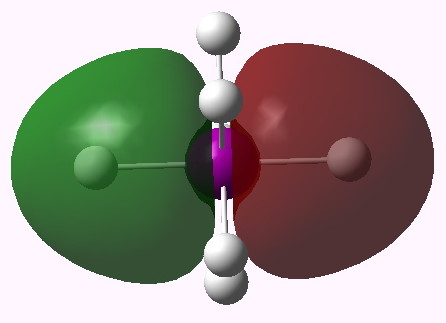MO 11 (A2'')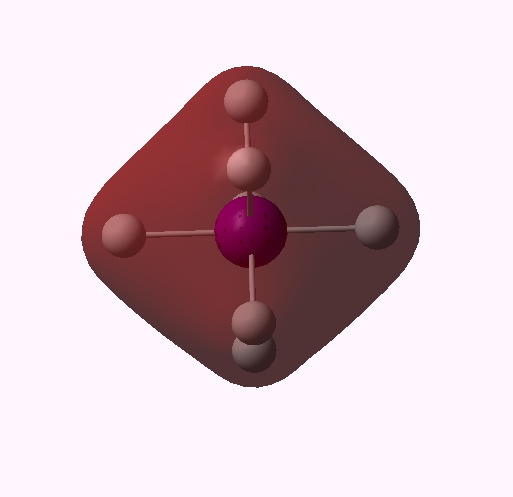MO 10 (A1')

One notices that whilst orbitals 10-14 are clearly bonding in the I-H region, orbitals 15-16 seem antibonding in that region (there is a node along the I-H bond). We are seeing much the same phenomenon that occurs when the bond order of 3 in N2 is reduced to 1 in F2 due to occupancy of anti-bonding orbitals. Can this be quantified? NBO (5.9) analysis reveals the following.

1. The effective (pseudopotential) core has 28 electrons, and the outer core 18. The valence orbitals contain 13.79, and only 0.21 electrons are Rydberg (higher shell). So little occupancy of e.g. 6s/6p then!
2. The Wiberg bond index indicates each H has a total bond order of very close to 1 (its natural valence state), whilst I is 3.45. Remember that the maximum total bond index of a covalently bound atom using a pure octet of valence electrons is 4 (8/2, think carbon). The iodine is NOT hypervalent!
3. So why, if its not hypervalent, is it so strongly hypercoordinate? Well, there are 14 valence electrons, but they do NOT all occupy the I-H regions, which have bond orders between 0.46 (equatorial) or 0.57 (axial). The only other place they can be is in the H-H regions! Consider the bond order values between say hydrogen 2 to the four other equatorial atoms 4,5,7,8. They are respectively 0.05, 0.15, 0.05, 0.15. So each hydrogen has a total bond order of 1, but only slightly more than half of this comes from the I-H, the rest comes from H-H. To put it another (approximate) way, of the 14 valence electrons, ~8 might be considered to be associated with I-H bonds, and ~6 with H-H bonds. The hypervalency has been in effect absorbed into the H-H regions. This means no atom in this molecule is at all hypervalent.
4. Well, if the iodine is not hypervalent, and some of its valence electrons occupy I-H anti-bonding orbitals, why is it stable at all (in the sense that all the vibrations are real, and it’s clearly a minimum in the potential energy surface). Here, I merely speculate. Iodine has a large core charge, and hence the inner core electrons are starting to exhibit relativistic contractions. This effect stabilizes the outer 5s/5p electrons, and so occupancy of  anti-bonding MOs generated from such AOs is not so unfavourable as one might expect. Perhaps, the  hypercoordination shown by  IH7 is after all a relativistic effect rather than a hypervalent effect?  In which case, what will  AsH7 show?

So, what might have been an archetypal covalent hypervalent molecule is no such! IH7 shows entirely normal valencies, one for H and the iodine does not even reach four (if anything, its sub-valent rather than hypervalent). One may be entitled to ask if ANY main group element exhibits hypervalency!

### 5 Responses to “Hypervalency: Is it real?”

1. […] Henry Rzepa Chemistry with a twist « Hypervalency: Is it real? […]

2.Henry Rzepa says:

In the post, I mooted that relativistic effects might contribute to the properties of IH7, and that AsH7 might enhance these. Thus MO 10 above is mostly 1s/5s in character, and the s shells are the most affected by relativistic contractions. Indeed, the orbital energy with I is -0.842 au, but the corresponding As molecule with a 6s contribution is actually lower at -0.888 au. The 5p/6p energies are more similar, since here the p-AO is less contracted; MO 11 is -0.494 vs -0.488. MO16 (with a lot of s-AO) is -0.232 vs -0.235 au. In this sense, the bonding valence electrons in AsH7 tend to be more stable than those in IH7, despite being in a higher (6 vs 5) valence shell. So the relativistic effect does seem quite strong.

BrH7 on the other hand shows the match for MO 10 to be -0.951, since the 4s/4p shell has a radial distribution much closer to the nucleus. It however is destabilized by a shorter H…H distance (~1.92Å), compared to 2.09 for IH7 and 2.23Å for AsH7. The optimum van der Waals distance for H…H contacts is ~2.2Å, so only the latter does not suffer any formal steric repulsions. In the case of BrH7, the total Wiberg bond index around the Br is 3.19 (the hydrogens are 1.004), so even more electrons have fled from the Br-H bonds into the H…H regions. Clearly, thermodynamically, all this H…H density is only encouraging H2 molecules to form, which is what would happen in reality, and which is why these higher halogen hydrides have in fact never been observed (although it is worth noting that BrH7 IS a minimum on the potential energy surface).

3.Paul says:

While you argue that hypervalency may not occur it is now a commonly accepted theory. X-ray analysis (which in my view is much more reliable and accurate than DFT calculations) show that the bond length between the iodine centre and its “ligand” (most commonly an electronegative halide) are shorter than a purely ionic bond would be.

•Henry Rzepa says:

If the theory of hypervalency is commonly accepted, than species such as IH7 certainly contradict the most common definition of hypervalency, which is octet (in the case of main group elements) expansion of the valence shell. That definition is clearly not borne out by analysis of the wavefunction. The only way out is to allow each hypervalent bond to be defined by less than a Lewis electron pair. However, that seems synonymous with hypercoordination. We would then be left with the very undesirable situation where the definition of hypervalency and hypercoordination cannot be separated and would have to be treated as synonyms. In effect, they already are, since many references to hypervalency in the literature probably actually mean hypercoordination. Let me give one example (DOI: 10.1021/ja710423d) of the ambiguity between hypervalence and coordination. In the title of this article, hexacoordinate is used, but the main body of the text discusses hypervalence instead. In the references to this article, a precedent is cited (ref 6) as DOI: 10.1021/ja043802t for which the title uses the term hypervalent rather than hypercoordinate, although the type of interaction being considered is the same in both cases.

As to the bond lengths, well bonds defined as hypercoordinate may still be significantly covalent, and indeed shorter than a purely ionic bond (however that might be defined outside of an ionic lattice). A considerably under-used (probably because its difficult) technique is to employ x-ray crystallography to measure electron densities in bonds rather than at atoms. This can, with a bit of effort, result in topological analyses of e.g. ρ(r) and the Laplacian, which can directly probe the properties of the electrons in the bond. But for the vast majority of molecules, we do not have this information. It is therefore not immediately obvious if merely measuring a bond length will invariably tell us if the atom it is connected to is hypervalent (in the octet sense) or not.

Oh, I forgot to mention that a CCSD calculation on IH7 gives an almost identical analysis. Where CCSD has been calibrated against accurate X-ray measurements, it agrees almost perfectly (see http://www.ch.ic.ac.uk/rzepa/blog/?p=2251, although that does have a twist in its tail which suggests it’s the experiment rather than theory that is wrong!).

4.Henry Rzepa says:

One further thought about hypervalency vs coordination. In a previous post, I discussed the structure of solid carbon dioxide. It is clearly hexa-coordinate, in which two of the bonds are clearly strong, and four are clearly weak. This sort of interaction is often described as hypervalent. Should it be? Even in PCl5, three bonds are stronger than the other two, but what is the difference between these two molecules? I argue none, since neither is hypervalent.